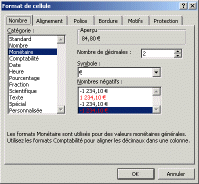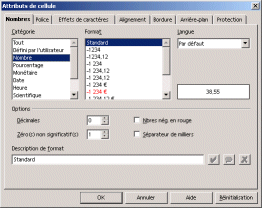## Cell Content

A cell of a worksheet can contain a value or be empty. The value of a cell has two essential characteristics:

• a type, which means the intrinsic type of the data. There are generally three types of values:
• numeric values, for example 3.1415927, 58%, or 1984.
• alphanumeric values, for example Table2, Title or Name.
• formulas, which means expressions that represent calculations based on data and performed by the spreadsheet.
• a format that represents the way the spreadsheet displays the data. For example, if it is a number, the spreadsheet can be set to display it as an integer, as a decimal number (as well as the number of decimal places displayed), etc.
The cell may have a style, which means a particular visual appearance (font, size, color, border, etc.), independent of the value it contains.

## Numeric Data Entry

When entering numbers in a cell (including monetary signs, percentages, etc.), the spreadsheet interprets the data as being numbers, which makes the use of mathematical formulas on the data possible. By default (without action on your part), the data recognized as being numbers will be right justified in the cell.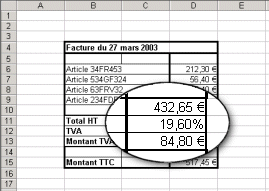## Alphanumeric Data Entry

When entering letters in a cell, the spreadsheet interprets the data as being alphanumeric, thus blocking the option of using mathematical tools on the data. By default (without action on your part), the data recognized as being alphanumeric will be left justified in the cell.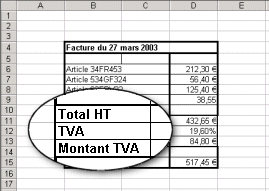## Entering a Formula

Formulas are expressions that, when they are interpreted by the spreadsheet, allow calculations to be performed using data present in other cells.

To enter a formula in a cell, select the cell and start entering the formula starting with the "equal" sign (=)!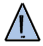If the syntax of the formula entered after the equal sign (=) is not correct, the spreadsheet will display an error message preceded with the # character! In case of an invalid cell reference, the spreadsheet will display the #REF! value.

A formula may contain references to other cells, expressions, which means operators that allow simple operations to be performed on the values of other cells, as well as functions.

The integrated functions of the spreadsheet allow elaborated calculations to be performed based on values in the spreadsheet, like averages, rounding, etc.

## Defining the Format of a Cell

The format of a cell defines the way its value will be displayed by the spreadsheet. To define the format of a cell, click on the cell, go to the menu Format > Cell and choose the Number tab (or Numbers in StarOffice). The spreadsheet will offer a series of formats divided by category:

 Excel StarOffice